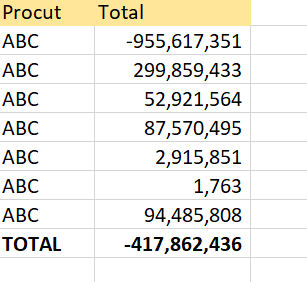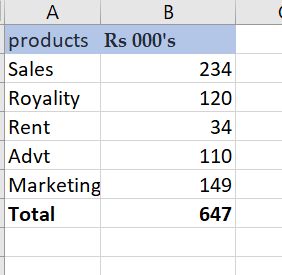# Compare Two excel file total value

Hi,
i have two excel files , compare master.xlsx total value to consolidate.xlsx total value . but problem is here the data in consolidate file the values in lacks(000).
how to check it ?
master.xlsx (9.6 KB)
consolidate.xlsx (9.7 KB)1 Like

Hi

May be i will tell you the way to get those two total values alone in two different variables
Based on which you can check or decide on how to compare them

For both the excel use these steps

—use a excel application scope and pass the file path as input and get the datatable with read range activity and name the output as dt

—then use a ASSIGN ACTIVITY like this

Str_total_1 = dt.AsEnumerable().Where(Functiona(a) a.Field(of String)(“firstcolumnname”).ToString.ToUpper.Contains(“TOTAL”))(0)(“your second columnname”).ToString

This will give the total value

Use the same expression for both th excel with corresponding column name

Later we can convert this to int32 by this expression in assign activity

int_total = Convert.ToInt32(Str_total_1.ToString)

Cheers @Anand_Designer

Cheers @Anand_Designer

Total 1:

``````total1 = Convert.ToInt32(dt.AsEnumerable().Where(Function (row) row.Field(of String)("Procut").ToString.ToLower.Contains("total"))(0)("Total"))
``````

Total2:

``````total2 = Convert.ToInt32(dt2.AsEnumerable().Where(Function (row) row.Field(of String)("products").ToString.ToLower.Contains("total"))(0)("Rs 000's").ToString + "000")
``````